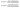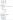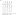HCF – LCM

Why is H.C.F and L.C.M important?
This is the very basic concept from numbers which is useful in the chapters of time and distance, time and work, pipes and cisterns, etc. The tricks used to find
L.C.M and H.C.F of two or more numbers help in finding out quick solutions and thus reduce time during examinations.
The concept of L.C.M. is important to solve problems related to racetracks, traffic lights, etc.
The applications of H.C.F. are calculating the largest size of tile, the largest tape to measure the land, the maximum scale to measure length etc.

Important terms:
1) Factors: Factor is a number which exactly divides another number.
A is said to be a factor of B if it completely divides B.

Example: 3 and 4 are factors of 12

2) Multiple: A multiple of a number, is the number which is exactly divisible by other number. B is said to be a multiple of A if it is completely divisible by A.
Example: 12 is a multiple of 4

3) Common factor: A common factor of two or more numbers is a number which exactly divides each of them.
Example: 7 is a common factor of 14, 56, 98……..

4) Common multiple: A common multiple of two or more numbers is a number which is exactly divisible by each of them.
Example: 24 is a common multiple of 2, 3, 4, 6, 8 and 12

4) H.C.F/G.C.F: (Highest Common Factor / Greatest Common Factor). H.C.F of two or more numbers is the greatest among all the common factors of them.

5) L.C.M.: (Lowest common multiple). The LCM of two or more numbers is a least among all the common multiples of the numbers.

H.C.F. and L.C.M. of FractionsImportant results on HCF – LCM:

• Product of two numbers = Product of L.C.M and H.C.F.
• HCF of numbers also divides their differences.

Methods to find HCF of numbers:

Factorization Method:

Ex. Find the HCF of 156, 244 by factorization method.LCM (189, 225) = 3 × 3 × 21 × 25 = 4725

Examples:

Ex.1    The HCF of two numbers is 13 and the other two factors of their LCM are 11 and 14. The smaller of two is:

1. a) 113 b) 143 c) 242              d) cannot be determined

Sol.:     Let the numbers be 13x and 13y.

Then LCM (13x, 13y) = 13 × 11 × 14.

So, x = 11 and y = 14

Smaller no. = 13 × 11 = 143

Ex.2    Six bells commence tolling together at the intervals of 3, 6, 9, 12, 15 and 18 respectively. In 30 minutes, do they toll together?

1. a) 10 b) 15 c) 11                d) 18

Sol.:     LCM (3, 6, 9, 12, 15, 18) = 180

So, all the bells will toll together after every 180 seconds.

Total time = 30 minutes = 1800 seconds

No. of times all the bells toll together = (1800/180) + 1 = 11

Ex.3    Find the greatest number of 4-digit which leaves remainder of 3 when divided by 8, 16, 24, 48.

1. a) 9987 b) 9984 c) 9848            d) 9948

Sol.:     The number which leaves no remainder on dividing by 8, 16, 24 and 48 is their LCM.

So, LCM (8, 16, 24, 48) = 48

The greatest 4-digit multiple of 48 is 9984 which is completely divisible by all the given numbers.

So, the greatest number of 4-digit which leaves remainder of 3 = 9984 + 3 = 9987

Ex.4    Product of two numbers is 16464. If their LCM is 1176, find their HCF.

1. a) 18            b) 25            c) 20                d) 14

Sol.:     Product of two numbers = LCM × HCF

So, 16464 = 1176 × HCF

HCF = 16464/ 1176 = 14

Ex.5    Determine the HCF of 5/18, 15/22, and 25/36.

1. a) 15/369             b) 75/11                 c) 5/396           d) 1

Sol.:     HCF of fractions = HCF of numerators / LCM of denominators

HCF    = HCF (5, 15, 25)/ LCM (18, 22, 36)

= 5/ 396

Ex.6    Find the difference between the HCF and LCM of 375, 500, 225 and 450.

1. a) 2275           b) 2225               c) 4525            d) 4475Ex.7    Which of the following cannot be the HCF of the numbers whose LCM is 160?

1. a) 32 b) 48 c) 80                d) 20

Sol.:     We know, HCF always divides LCM of numbers.

Here, 48 doesn’t divide 160.

So, 48 cannot be HCF of the numbers whose LCM is 160.

Ex.8    Find the greatest number that will divide 579 and 466 leaving remainders 11 and 10 respectively?

1. a) 16 b) 4 c) 8                  d) 12

Sol.:     Given numbers = 579, 466

Respective remainders = 11, 10

So, original numbers = 579 – 11 = 568 and 466 – 10 = 456

HCF (568, 456) = 8

Ex.9    The product of LCM and HCF of two numbers is a square of 25. If one of the numbers is 125, then what is the other number?

1. a) 15 b) 5 c) 425              d) None of these

Sol.:     Given, LCM × HCF = 252

One of the numbers = 125,

Let the other number be y.

We have,

LCM × HCF = Product of two numbers

625      = 125 × y

625/ 125     = y

Y         = 5

Ex.10  Find the greatest 3-digit number that is exactly divisible by 15, 25, 20 and 30.

1. a) 930 b) 990 c) 900              d) 887

Sol.:     A number exactly divisible by 15, 25, 20 and 30 is LCM of numbers.

LCM (15, 25, 20, 30) = 300

So, greatest 3-digit multiple of 300 is 900.

Ex.11  What will be the least number which when doubled will be exactly divisible by 14, 21, 24 & 35?

1. a) 420 b) 840 c) 1050            d) 630

Sol.:     A number exactly divisible by 14, 21, 24 and 35 is LCM of numbers.

LCM (14, 21, 24, 35) = 840

So, the required number is 840/2 = 420

Ex.12  The least number that should be added to 2578 so that the sum is exactly divisible by 5, 6, 4 and 10 is:

1. a) 11 b) 2 c) 35                d) 19

Sol.:     A number exactly divisible by 5, 6, 4 and 10 is LCM of numbers.

LCM (5, 6, 4, 10) = 60

Nearest multiple of 60 is, 2574.

So, the number which must be added to 2578 so that it will be divisible by 5, 6, 4 and 10 is (6 – 4) = 2

Ex.13  Three friends J, K and L jog around a circular stadium and complete one round in 12, 18 and 20 seconds respectively. In how many minutes will they all meet again at the starting point?

1. a) 5 b) 12 c) 3                  d) 8

Sol.: All three friends will meet again at the starting point in the time equal to LCM of 12, 18 &amp; 20.
LCM (12, 18, 20) = 180 seconds = 3 minutes.
So, J, K &amp; L will meet at the starting point in 3 minutes.

Ex.14  How many pairs of numbers are there such that their HCF and LCM are 12 and 924 respectively?

1. a) 1 b) 2 c) 3                  d) 4

Sol.:     Given, HCF = 12, LCM = 924

Let the numbers be 12x and 12y

We have,

LCM × HCF = Product of two numbers

12 × 924  = 12x × 12y

924/ 12 = xy

77        = xy

(x, y)    = (1, 77) or (x, y)         = (11, 7)

So, there are 2 pairs satisfying given conditions.

Ex.15  Let A be the greatest number that divides 3900, 4650 and 6325 leaving same remainder in each case. What is the sum of digits of A?

1. a) 7 b) 19 c) 22                d) 34

Sol.:     A         = HCF (4650 – 3900, 6325 – 4650, 6325 – 3900)

= HCF (750, 1675, 2425)

= 25

Ex.16  Three numbers are in a ratio 4: 5: 6 and their LCM is 1800. Find their HCF.

1. a) 10 b) 60 c) 12                d) 30

Sol.:     Let the numbers be 4x, 5x and 6x.

LCM (4x, 5x, 6x) = 1800

60x = 1800

So, x = 30

The numbers are 4 × 30, 5 × 30, 6 × 30.

HCF = 30

Ex.17  Product of two coprime numbers is 231. Their LCM is:

1. a) 1 b) 231 c) Equal to their HCF             d) Cannot be determined.

Sol.:     LCM of co-prime numbers = product of those numbers.

So, LCM of numbers = 231

Ex.18  Three cloths of 7m, 7.5 m and 9.5 m, respectively have to be cut into same length pieces of maximum size. What will be the number of pieces?

1. a) 24 b) 48 c) 120             d) 96

Sol.:     The maximum length of each piece can be the HCF of the given lengths.

HCF (7, 7.5, 9.5) = 0.5

Total number of pieces            = 7/0.5 + 7.5/0.5 + 9.5/0.5

= 14 + 15 + 19

= 48

Ex.19  Find the HCF of 0.63, 1.05 and 2.1.

1. a) 0.21 b) 0.44 c) 0.44             d) None of these

Sol.:     Let the numbers be 63, 105, 210        …. (Multiply each number by 100)

HCF (63, 105, 210) = 21

So, HCF (0.63, 1.05, 2.1) = 0.21        …. (Divide HCF by 100)

Ex.20  What is the least multiple of 8 which leaves a remainder of 4 when divided by 6, 9, 15 and 18?
a) 184              b) 150              c) 183              d) 178

Sol.:     A number completely divisible by 6, 9, 15 and 18 is LCM of these numbers.

LCM (6, 9, 15, 18) = 90

The required number is, 90k + 4.

For k = 1, the number is = 94              … (Not divisible by 8)

For k = 2, the number is = 184            … (Divisible by 8)